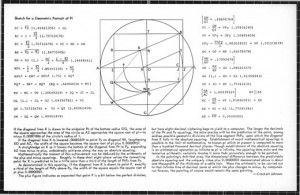# I am Pi: Thoughts on the Ratio of the Circumference of a Circle to Its Diameter

Every year, the celebration of Pi Day (March 14 is 3.14) grows more ambitious. Math teachers love to dream up unique classroom activities to celebrate Pi for it endless opportunity to calculate (3.14159265358989 and so on and so on.) This week Congress made it official. Tomorrow is National Pi Day.

I can't help but personally revel in this moment. I have a longtime association with the word, having been born and christened Beth Py (Lieberman came later with a wedding ring). The school yard playground was filled with bullies taunting me with insults (Py Face, Cow Pie).

But I found dignity in the Greek form of my name. I am Pi, the ratio of the circumference of a circle to its diameter.

Picking up the phone here at the Smithsonian, I set out to find out more about Pi and how it is represented in the national collections. Peggy Kidwell, the curator of mathematics at the National Museum of American History, graciously offered to be my guide offering me first, a unique mnemonic to recall the first of the chain of infinite digits in the number Pi. Simply count the number of letters in each of the words in this phrase, and you're off to a good start:

"How(3) I(1) want(4) a(1) drink(5), alcoholic(9) of(2... and so on) course, after the heavy chapters involving quantum mechanics (3.14159265358989)." (Now, that's fodder for a cocktail party.)

But here's a fact that will knock your socks off. You remember from childhood, Harold and the Purple Crayon, the peripatetic boy whose crayon drew him a world and a story? The author of that seminal storybook, Crockett Johnson did a series of paintings between 1966 and 1975 to represent Pi (above). Many of Johnson's paintings are in the collections at American History, and if you go to the museum today you can find other mathematical artifacts in the science and technology galleries.

For more on Pi Day, check out our companion blog, Surprising Science, tomorrow, on the actual holiday.

To explain his work, Johnson offers this treatise, which I am willing to post, but I'll leave the explanation to Kidwell, after the jump:Images courtesy of the National Museum of American History

"This oil painting on pressed wood, #52 in the series, displays one of Crockett Johnson’s original constructions. He executed this work in 1968. He was proud of the construction, and painted several other geometric constructions relating to squaring the circle. This construction was part of Johnson’s first original mathematical work, and was published in The Mathematical Gazette in early 1970. A diagram relating to the painting was published there.

To "square a circle" one must construct a square whose area is equal to that of a given circle using only a straight edge (an unmarked ruler) and compass. This is an ancient problem dating from the time of Euclid. In 1880, the German mathematician Ferdinand von Lindermann proved that pi is a transcendental number and that squaring a circle is impossible under the constraints of Euclidean geometry. Because this proof is complicated and difficult to understand, the problem of squaring a circle continued to attract amateur mathematicians like Crockett Johnson. Although he ultimately understood that the circle cannot be squared with a straight edge and compass, he managed to construct an approximate squaring.

The construction begins with a circle of radius one. In this circle Crockett Johnson inscribed a square. Therefore, in the figure, AO=OB=1 and OC=BC=√2 / 2. AC=AO+OC=1+ √(2) / 2 and AB= √(AC^2+BC^2) = √(2+√(2)). The artist let N be the midpoint of OT and constructed KN parallel to AC. K is thus the midpoint of AB and KN=AO - (AC)/2=(2- √2) / 4. Next, he let P be the midpoint of OG, and drew KP, which intersects AO at X. Crockett Johnson then computed NP=NO+OP = (√2)/4 + (1/2). Triangle POX is similar to triangle PNK, so XO/OP=KN/NP. From this equality it follows that XO = (3-2√(2)) /2. Also, AX = AO-XO =(2√(2)-1)/2 and XC=XO+OC=(3-√(2))/2. Crockett Johnson continued his approximation by constructing XY parallel to AB. It is evident that triangle XYC is similar to triangle ABC, and so XY/XC=AB/AC. This implies that XY=/2. Finally he constructed XZ=XY and computed AZ=AX+XZ=/2 which approximately equals 1.772435. Crockett Johnson knew that the square root of pi approximately equals 1.772454, and thus AZ is approximately equal to root(pi) - 0.000019. Knowing this value, he constructed a square with each side equal to AZ. The area of this square is AZ squared, or 3.1415258. This differs from the area of the circle by less than 0.0001. Thus, Crockett Johnson approximately squared the circle.